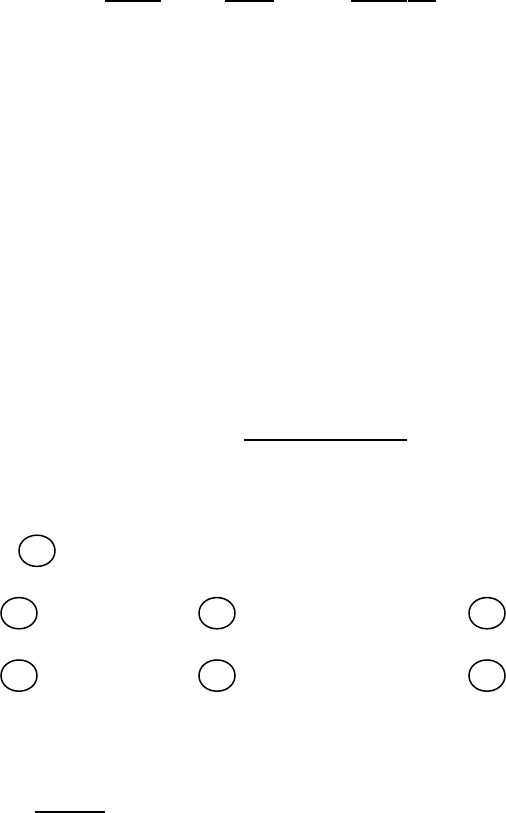• 二年级数学期中综合卷班别: 座号: 姓名: 一、口算（20分）4×6= 18÷6= 54+36= 30÷5= 350+250=95-82= 45÷9= 7×8= 54÷9= 2200+700= 49÷7= 84-38= 8×9= 66-15= 7300+1400= 54-35= 30+58= 28÷7= 3×7= 640-460=二、填空。(每空1分,共32分)1．24÷6=（ ），读作： 。除数是（ ）， 被除数是（ ），商是（ ）。2．在 里填上“＞”，“＜”或“=”。 239 293 999 1000 4千克 4000克 436 430 2750 2755 2500克 3千克3、算式: 4、按规律填数：（1）2060、2070、（ ）、（ ）（2）4010、4005、（ ）、（ ）5．将下列算式填在合适的（ ）里。 32÷8 7×6 72÷9 35+5 54÷6（ ）＞（ ）＞（ ）＞（ ）＞（ ）6． 右图有（ ）直角； 有（ ）锐角；
数学人教版二年级下：期中试题5

远山浅

108

8

3.0分

共4页 2021-05-17 免费班别
:
座号
:
姓名
:
20
4×6= 18
÷6= 54+36= 30÷5= 350+250=
95-82= 45÷9= 7
×8= 54
÷9=
2200+700=
49÷7= 84-38= 8
×9= 66-15= 7300+1400=
54-35= 30+58= 28
÷7= 3
×7=
640-460=
(
1
,
32
)
1
24÷6=
（ ），读作： 。除数是（ ），
被除数是（ ），商是（ ）。
2
．在 里填上“＞”，“＜”或“
=”
239 293 999 1000 4
千克
4000
436 430 2750 2755 2500
3
千克
3
算式
:
4
按规律填数：
1
2060
2070
、（ ）、（ ）
2
4010
4005
、（ ）、（ ）
5
．将下列算式填在合适的（ ）里。
32÷8 7×6 72÷9 35+5 54÷6
（ ）＞（ ）＞（ ）＞（ ）＞（ ）
6
右图有（ ）直角； 有（ ）锐角；
立即下载
评论(0)
没有更多评论了哦～

## 作者简介测试

暂未添加简介
文档
2336
合辑
0
文章
0